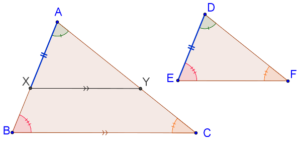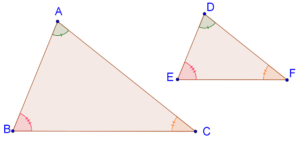# AA Criterion in Triangles

Go back to  'Similar-Triangles'

We have seen that if two triangles are similar, then they are equi-angular and their corresponding sides are proportional. We will now discuss the various criteria using which we can say that two given triangles are similar. The first of these is the AA criterion.

AA Criterion: Equi-angular triangles are similar.

This means that if the three angles of one triangle are respectively equal to the three angles of the other, then the two triangles will be similar. Ideally, the name of this criterion should then be the AAA criterion, but we call if the AA criterion because we need only two pairs of angles to be equal - the third pair will then automatically be equal.

Consider the following figure, in which $$\Delta ABC$$ and $$\Delta DEF$$ are equi-angular ( $$\angle A$$ = $$\angle D$$, $$\angle B$$ = $$\angle E$$ and $$\angle C$$ = $$\angle F$$ ):Using the AA criterion, we can say that these triangles are similar. This means that their sides will be proportional, that is:

$\frac{{AB}}{{DE}} = \frac{{BC}}{{EF}} = \frac{{AC}}{{DF}}$

Let us now discuss the proof of this criterion.

Proof: Consider the equi-angular triangles, $$\Delta ABC$$ and $$\Delta DEF$$ once again, such that $$\angle A$$ = $$\angle D$$, $$\angle B$$ = $$\angle E$$ and $$\angle C$$ = $$\angle F$$. Assume that AB > DE. Take a point X on AB such that AX = DE. Through X, draw segment XY parallel to BC to meet AC at Y, as shown below:Since XY || BC, we note that $$\Delta ADE$$ ~ $$\Delta ABC$$. Now compare $$\Delta AXY$$ with $$\Delta DEF$$:

1. $$\angle A$$ = $$\angle D$$ (given)

2. $$\angle AXY$$ = $$\angle E$$ (how?)

3.  AX = DE (by our choice of the point X)

By the ASA criterion, the two triangles are congruent. What does this mean? $$\Delta DEF$$ is congruent to $$\Delta ADE$$, which in turn is similar to $$\Delta ABC$$. Thus, $$\Delta DEF$$ is similar to $$\Delta ABC$$. This completes our proof.

Learn math from the experts and clarify doubts instantly

• Instant doubt clearing (live one on one)
• Learn from India’s best math teachers
• Completely personalized curriculum